Function Repository Resource:

# RandomMixedGraph

Change an undirected graph into a mixed graph

Contributed by: Peter Burbery
 ResourceFunction["RandomMixedGraph"][{n,m},frac] creates a random graph with n vertices and m edges, with frac of these as directed edges and the rest undirected. ResourceFunction["RandomMixedGraph"][{n,m},frac,spec] creates a table of random graphs with dimensions given by spec.

## Details

A mixed graph is one with both directed and undirected edges. ResourceFunction["RandomMixedGraph"] produces a mixed graph by making a random graph and converting a fraction of undirected edges into directed edges.
frac should be a number between 0 and 1 that determines the fraction of undirected edges which are coverted to directed edges. A frac value of 0 returns the original graph, while a frac value of 1 produces a fully-directed graph with randomly-oriented edges. A frac value of 0.5 would make approximately 50% of the edges directed.

## Examples

### Basic Examples (7)

Generate a random mixed graph with 20 nodes (vertices) and 48 edges, with a fraction of 0.75 arcs (edges):

 In:=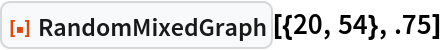Out=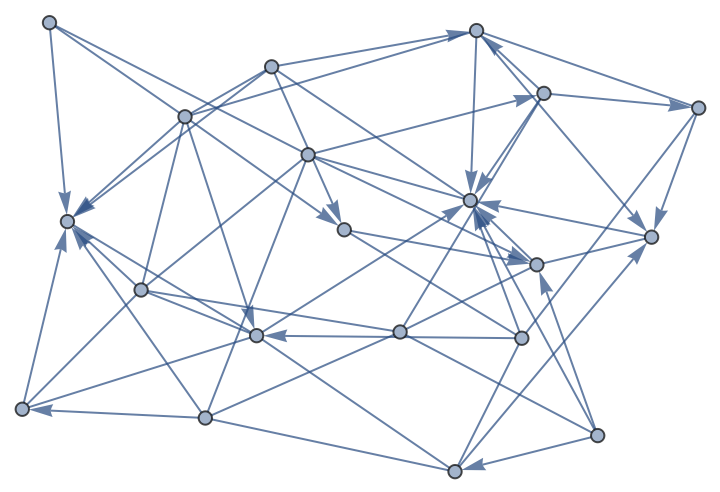Generate a graph with 0.5 directed edges:

 In:=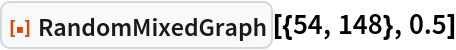Out=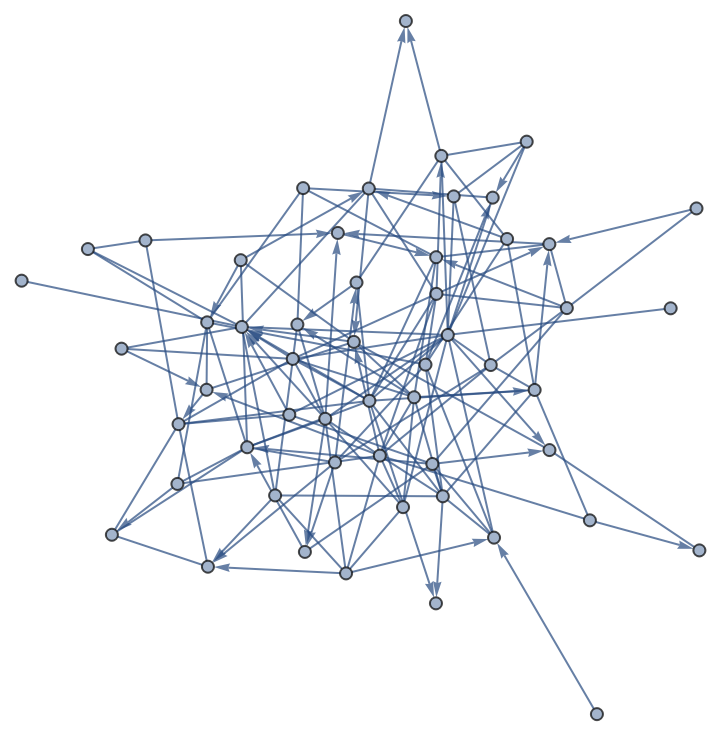Generate a list of random mixed graphs:

 In:=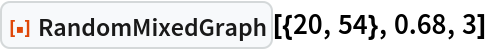Out=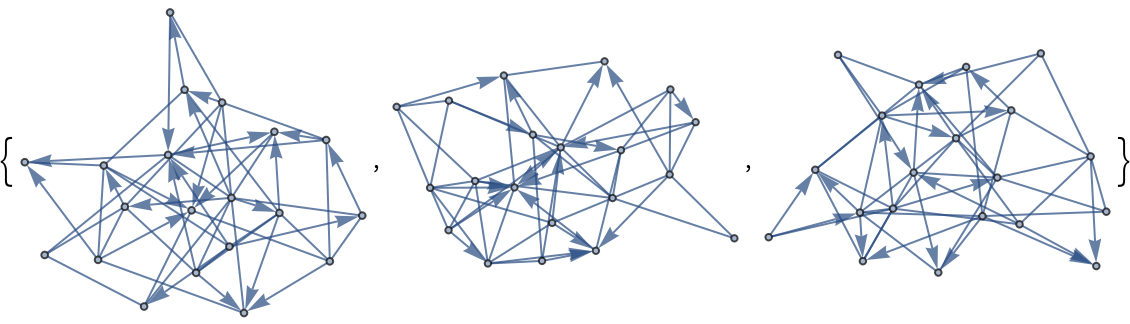Generate an array of mixed graphs:

 In:=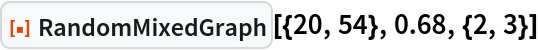Out=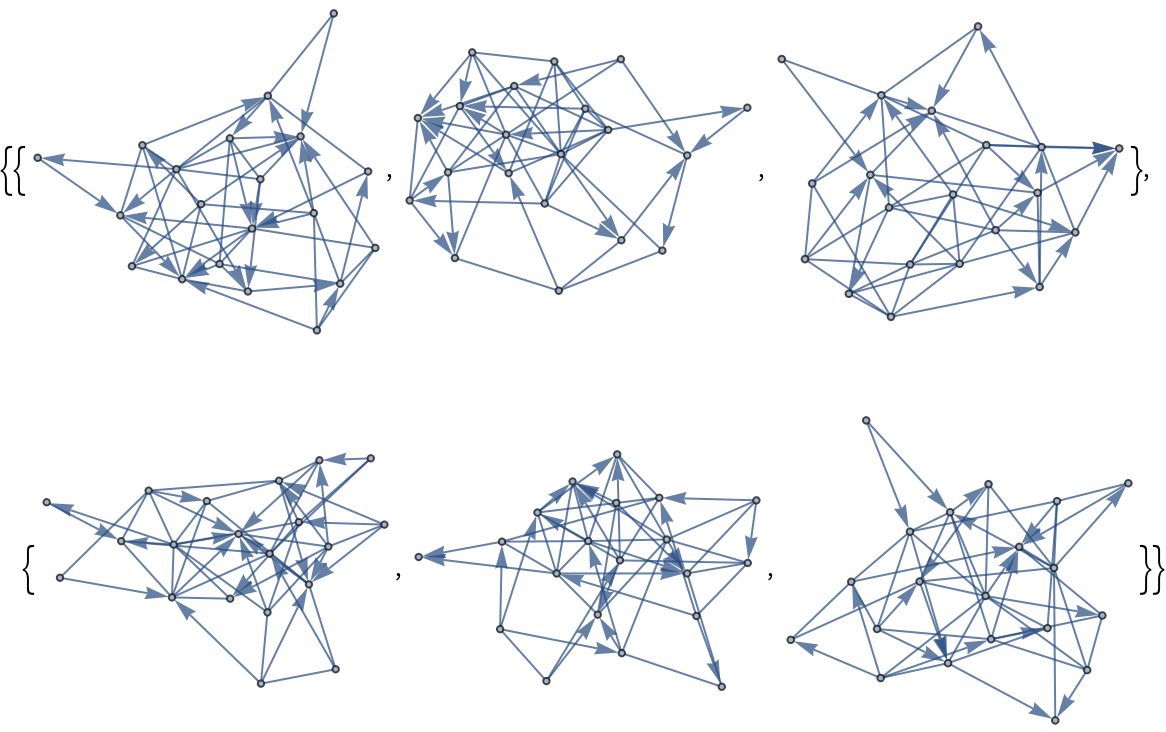Generate a random spatial graph with 148 nodes and 0.68 directed edges:

 In:=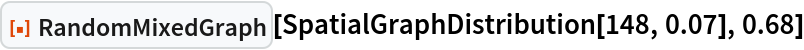Out=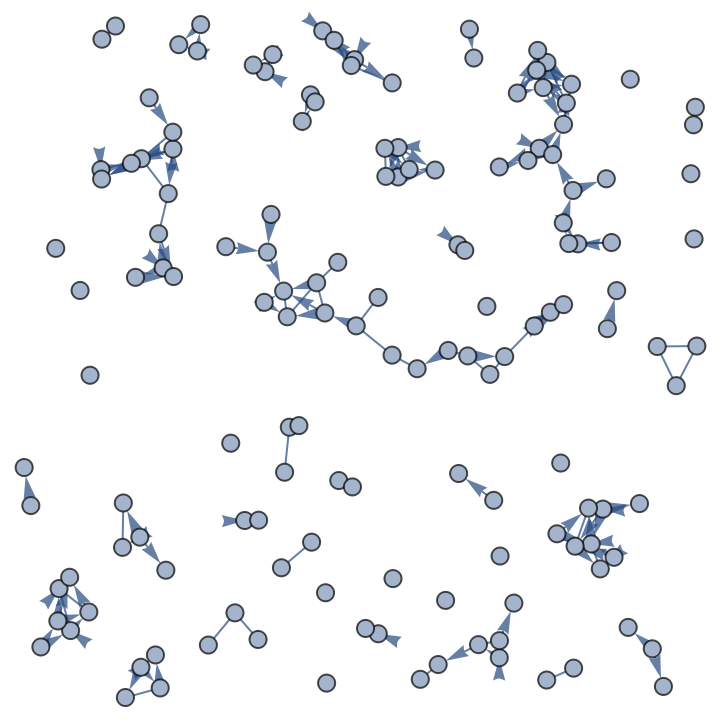Generate a list of random mixed graphs with the Barabasi-Albert graph distribution:

 In:=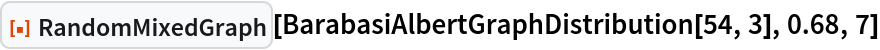Out=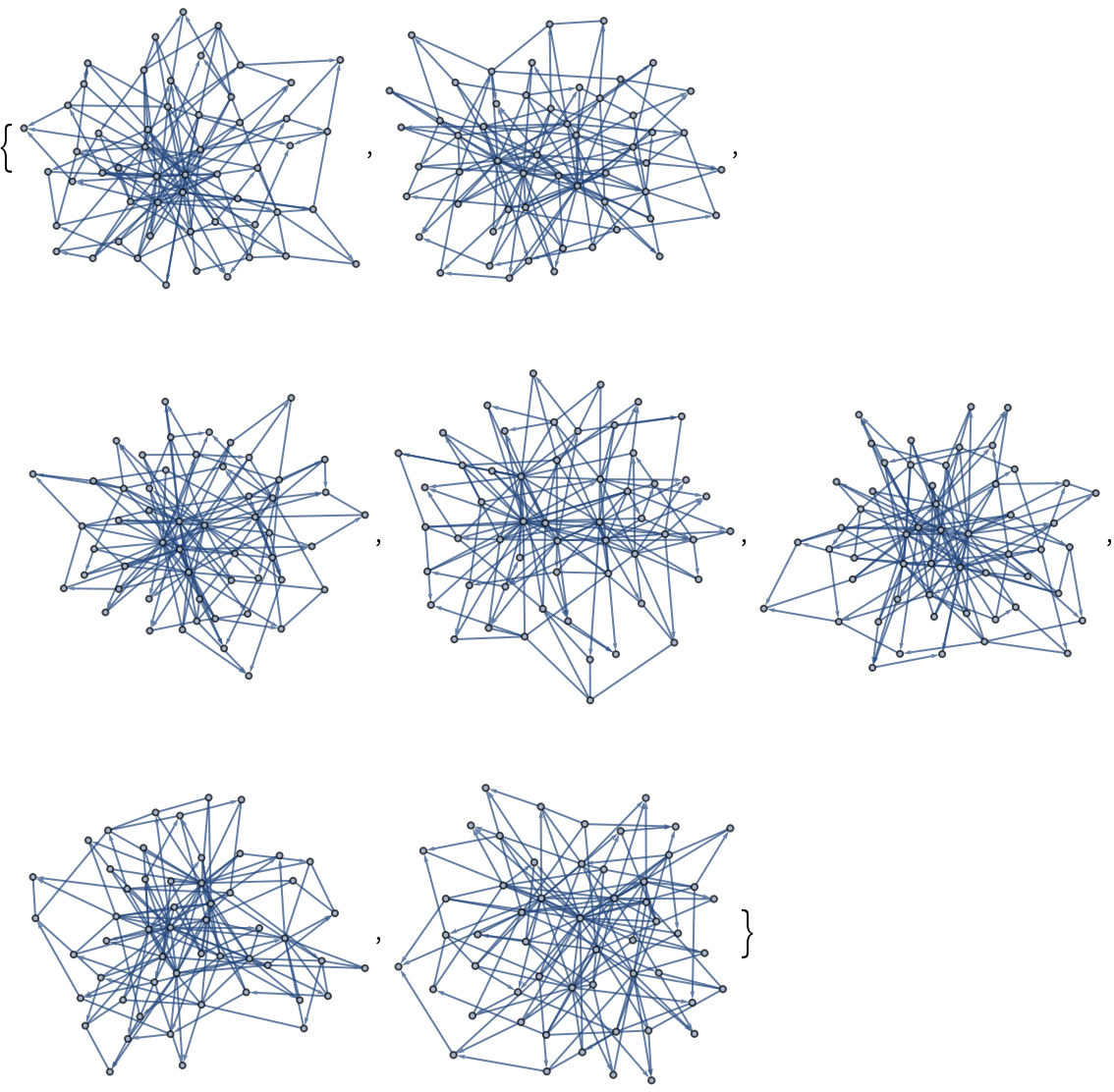Generate a 2x3 array of random graphs based on PriceGraphDistribution:

 In:=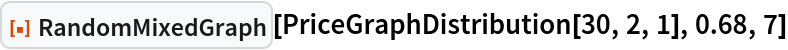Out=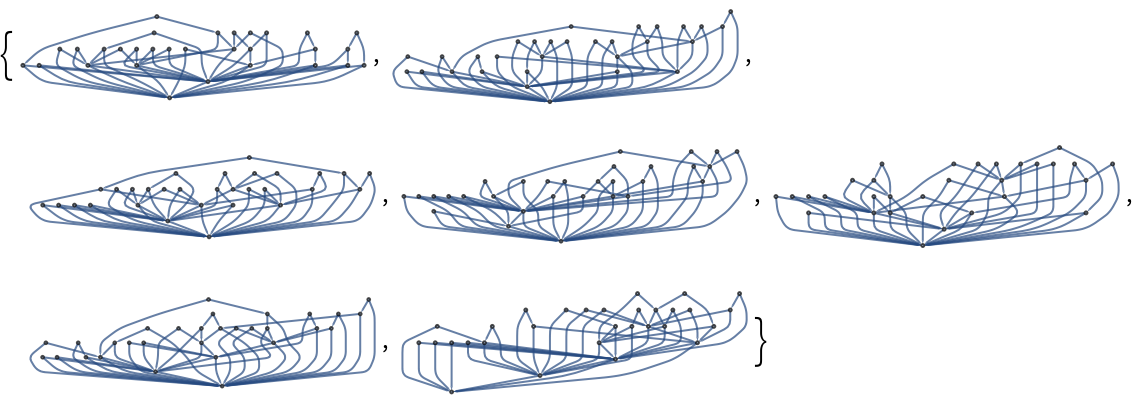### Applications (10)

Make a big mixed graph:

 In:=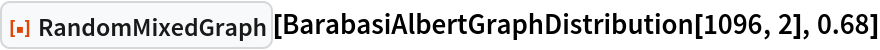Out=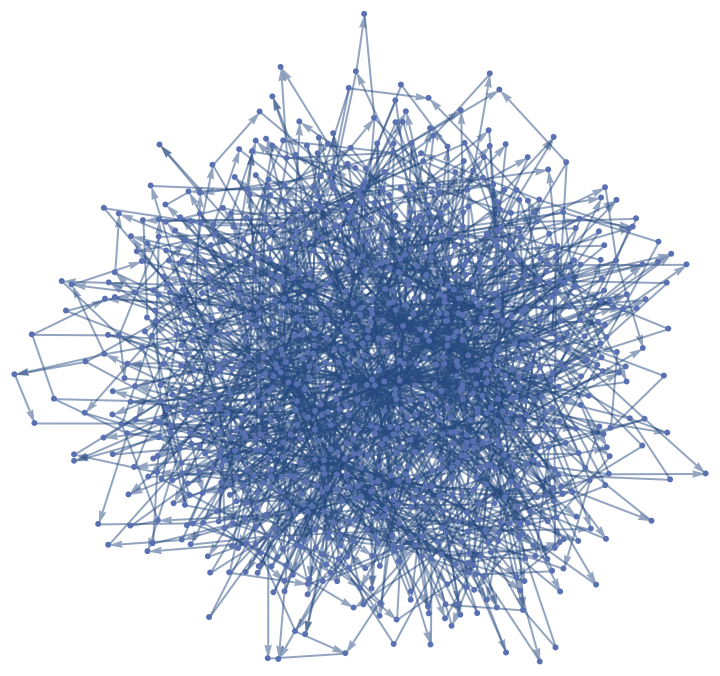Evaluate if a mixed graph has a Hamiltonian cycle:

 In:=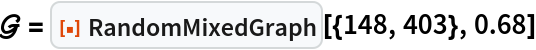Out=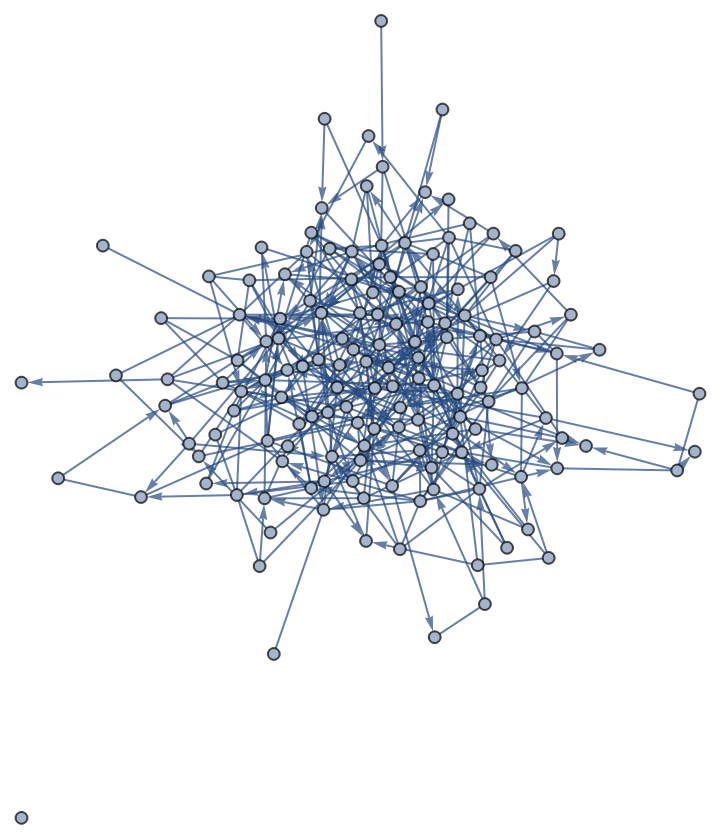In:=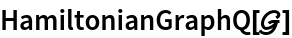Out=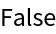Find the graph union of two mixed graphs:

 In:=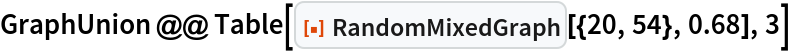Out=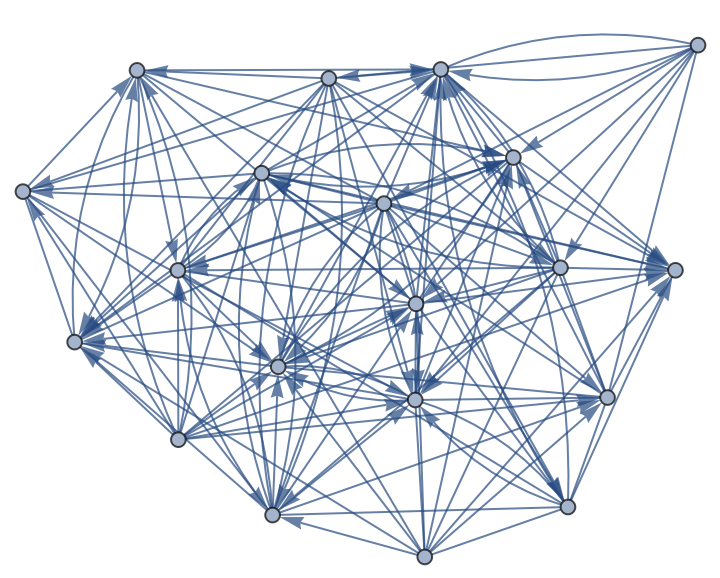Make an indexed mixed graph:

 In:=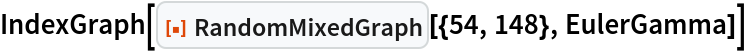Out=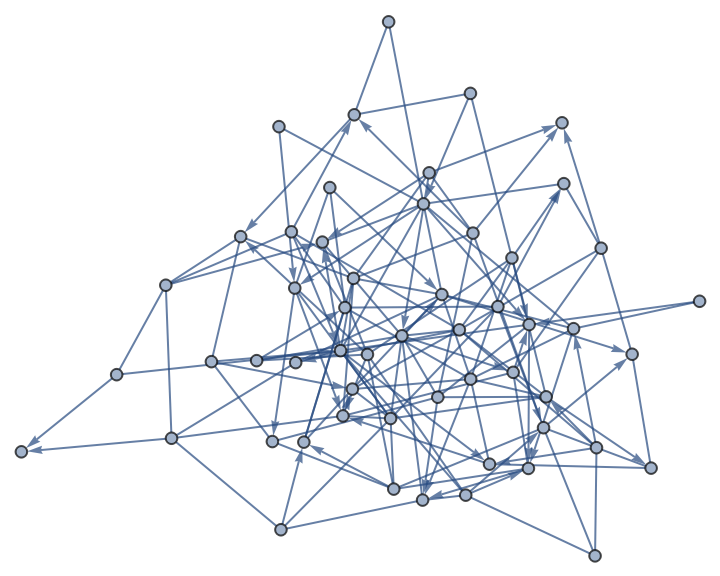Reverse the directed edges of a mixed graph:

 In:=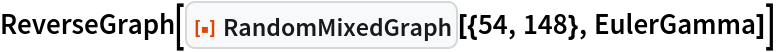Out=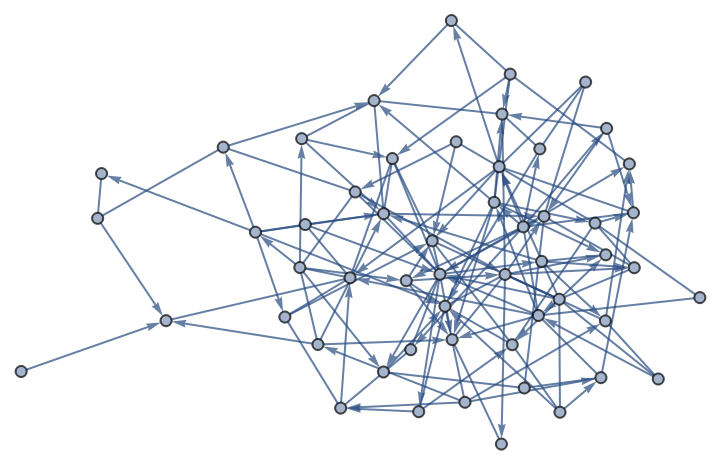Compute the graph product for various definitions for two mixed graphs:

 In:=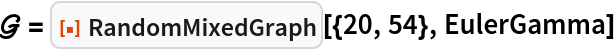Out=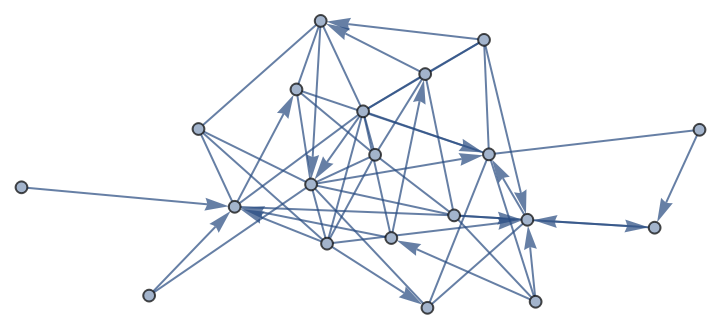In:=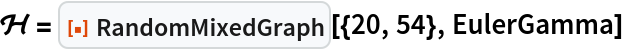Out=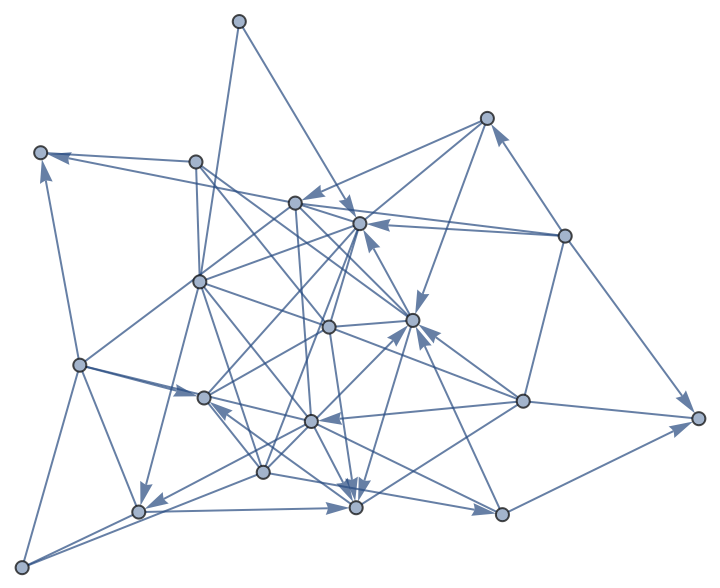The graphs can be very very big:

 In:=Out=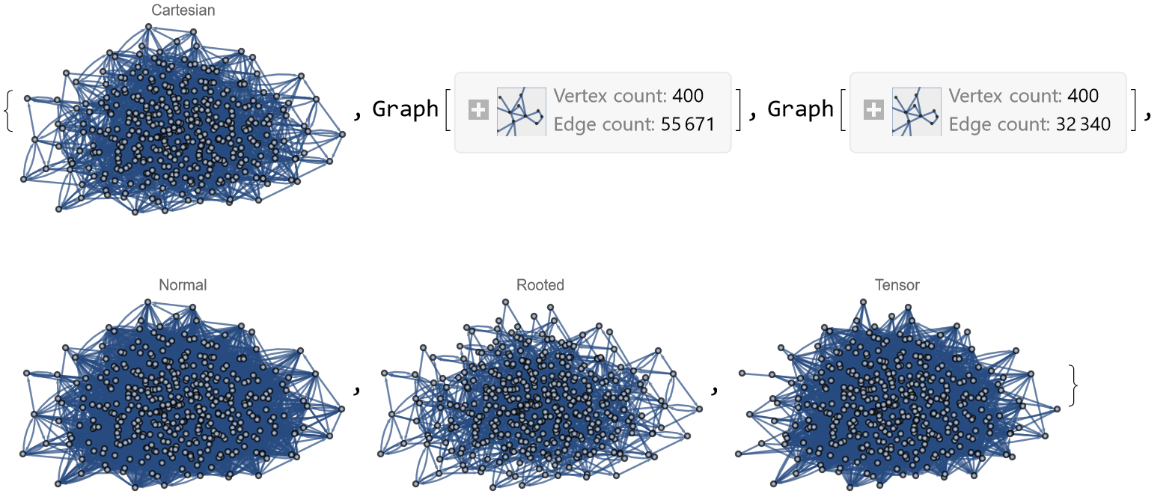Apply binary graph operations to two small mixed graphs:

 In:=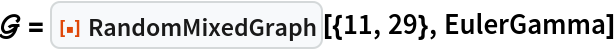Out=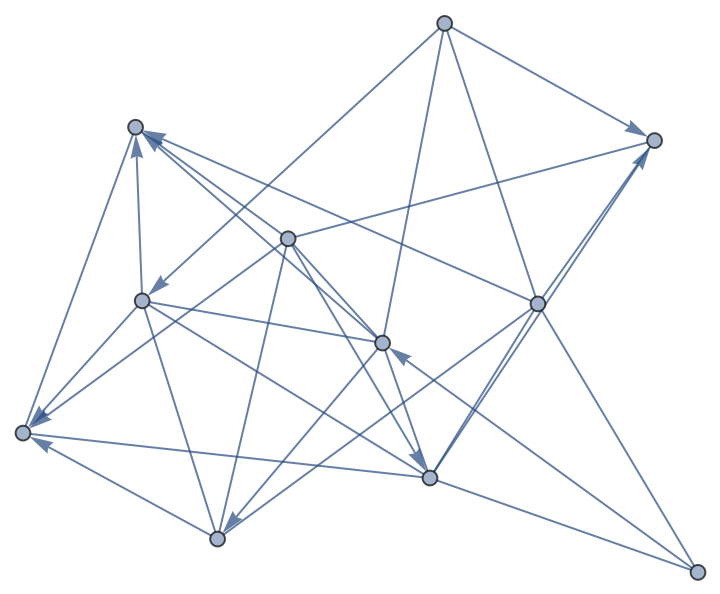In:=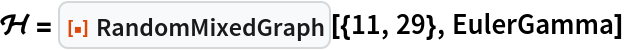Out=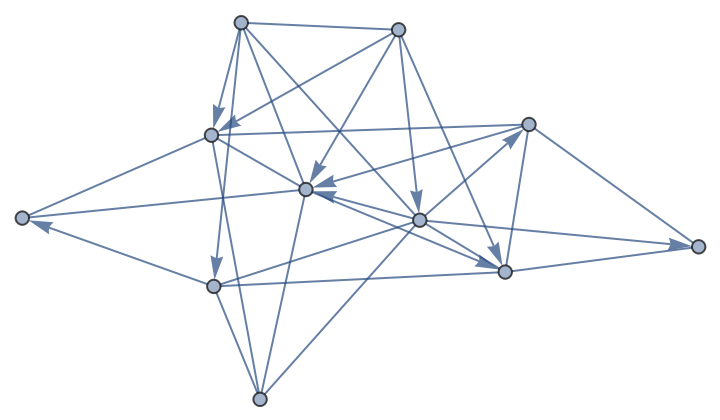In:=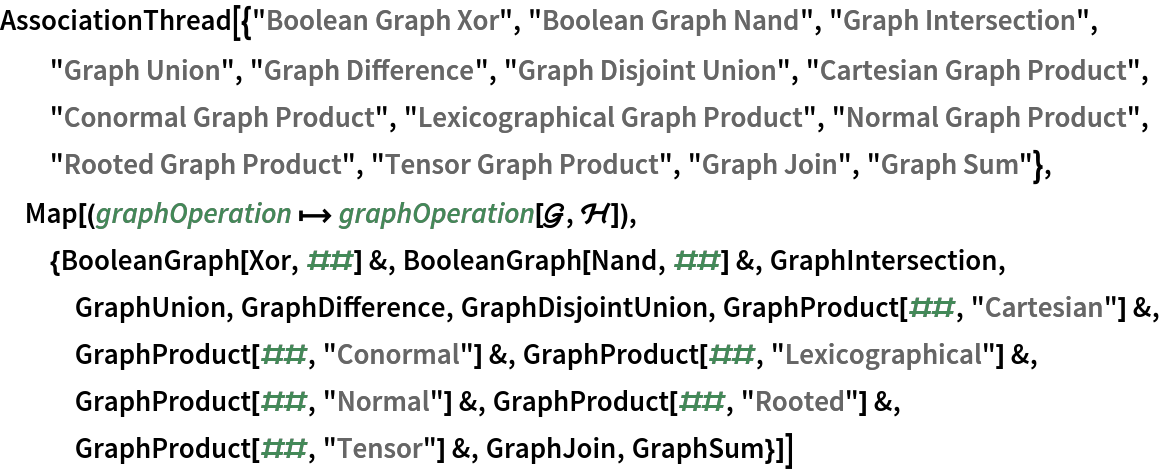Out=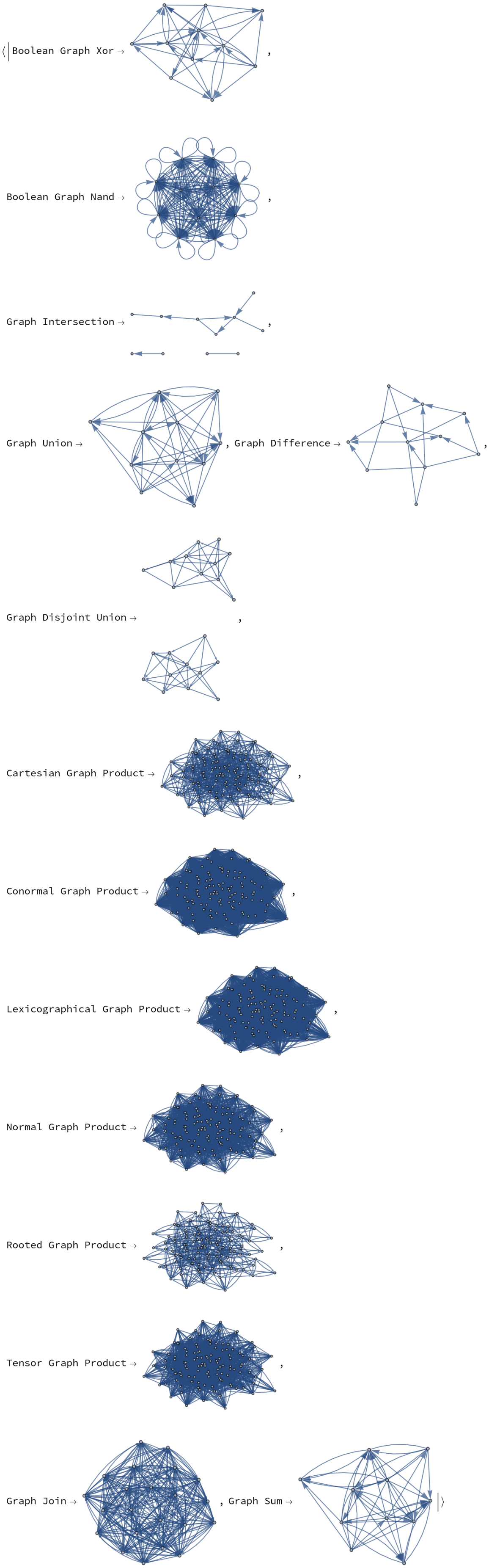Compute unary graph operations on a large random mixed graph:

 In:=Out=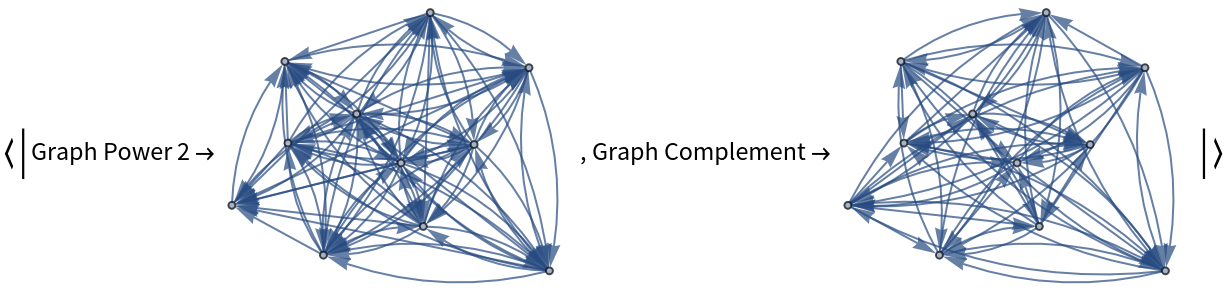### Properties and Relations (1)

Verify the output of the function produces a mixed graph:

 In:=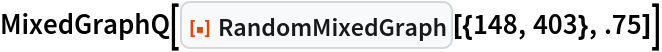Out=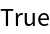Peter Burbery

## Version History

• 1.0.0 – 25 July 2022